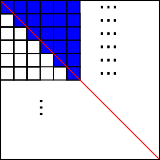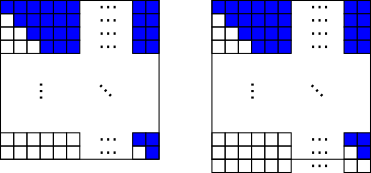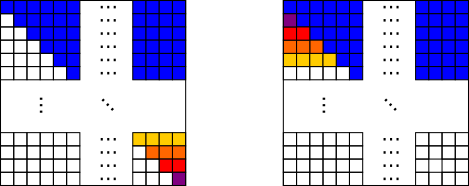You may also likeConsecutive Numbers

An investigation involving adding and subtracting sets of consecutive numbers. Lots to find out, lots to explore.Calendar Capers

Choose any three by three square of dates on a calendar page...Days and Dates

Investigate how you can work out what day of the week your birthday will be on next year, and the year after...

Square Grid

Age 11 to 14 ShortChallenge Level

Answer: $\frac{101}{200}$

Notice that there is a total of $100\times100=10 000$ little squares in the grid.

Cutting the grid in halfThe red line cuts the grid in half.

On each row, there is half of a shaded square on the 'wrong' side of the red line.

So it is $\frac12$ shaded plus $\frac{\frac12}{100}=\frac1{200}$ for the half square per line.

$\frac12 + \frac{1}{200} =\frac{101}{200}$

Add one more row so that the new shape is half shaded:Grid on right: $100\times101=10 100$ squares, $10 100\div2=5050$ are shaded.

Grid on left: also $5050$ shaded, out of only $10 000$
$\therefore$ fraction shaded is $\frac{5050}{10000}=\frac{101}{200}$

'Counting' the number of shaded squares by making full rows
Move shaded squares to make full rows (and empty rows):$1$ square is moved into the row with $99$ shaded,
$2$ into the row with $98$ shaded
...
$49$ are moved into the row with $51$ shaded
$50$ are left in the row with $50$ shaded

Total $50\times100+50=5050$ shaded squares (including the row which already had $100$ shaded)

Fraction shaded: $\frac{5050}{10000}=\frac{101}{200}$

You can find more short problems, arranged by curriculum topic, in our short problems collection.